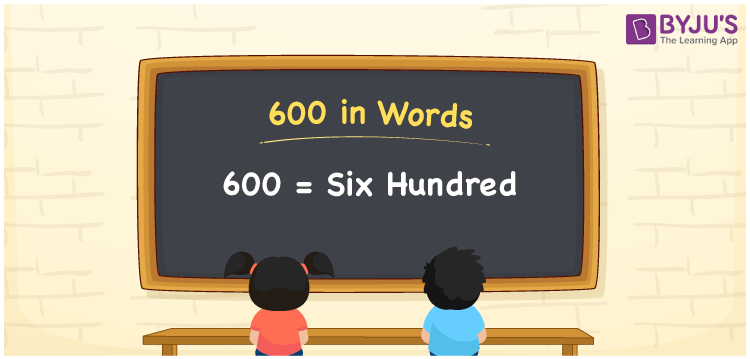# 600 in Words

600 in words can be written as Six Hundred. The concepts like counting numbers which are important from primary education are discussed here in brief. For instance, if you spend Rs. 600 in buying a T-shirt, then you can say that “I bought a T-shirt for Six Hundred Rupees”. The English alphabet is used to write 600 in words. Students can get a clear knowledge of numbers in words with the help of the study materials given at BYJU’S. The number 600 can be read as “Six Hundred” in English.

 600 in words Six Hundred Six Hundred in Numbers 600

## 600 in English Words## How to Write 600 in Words?

Place value chart and expanded form of 600 can be learnt easily in this section. There are three digits in the number 600. The place value chart for 600 is provided below for reference to help students understand the concepts effectively.

 Hundreds Tens Ones 6 0 0

The expanded form of 600 is provided below:

6 × Hundred + 0 × Ten + 0 × One

= 6 × 100 + 0 × 10 + 0 × 1

= 600

= Six Hundred

Hence, 600 in words is written as Six Hundred.

600 is a natural number that precedes 601 and succeeds 599.

600 in words – Six Hundred

Is 600 an odd number? – No

Is 600 an even number? – Yes

Is 600 a perfect square number? – No

Is 600 a perfect cube number? – No

Is 600 a prime number? – No

Is 600 a composite number? – Yes

## Frequently Asked Questions on 600 in Words

Q1

### How can 600 be written in words?

600 can be written as “Six Hundred” in words.
Q2

### Is 600 an odd number?

No, 600 is not an odd number because it is divisible by 2. 600/2 = 300
Q3

### Write Six Hundred in numbers.

Six Hundred can be written in numbers as 600.